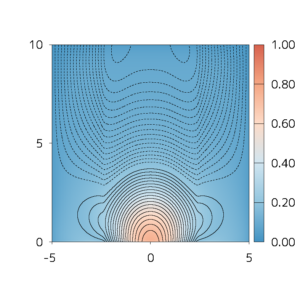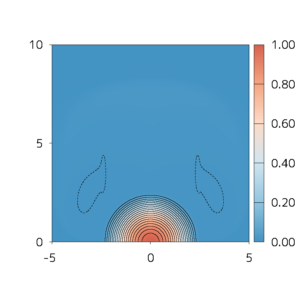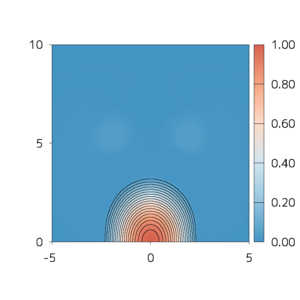# Science Nuggets No.27 (20200304)Numerical modeling of solar atmospheric magnetic fields including pressure and gravity

Takahiro Miyoshi (Hiroshima University)

Explosive phenomena in the solar corona such as solar flares and coronal mass ejections are considered to be processes to liberate free magnetic energy in the corona. In order to clarify and predict these phenomena, information on the three-dimensional distribution of the magnetic field in the corona is required. High-accuracy and high-resolution data of the magnetic field, however, cannot be directly measured except for the vector magnetic field on the photosphere. Thus, various methods for coronal magnetic field reconstruction from the photospheric magnetic field have be investigated.

Since the plasma pressure is much lower than the magnetic pressure in the corona, we expect that the coronal magnetic field can be approximated by a force-free magnetic field where the Lorentz force is zero. Accordingly, attention has been focused mainly on the development of numerical extrapolation techniques of the force-free field. The photospheric magnetic field, however, is not force-free in general because the effect of the plasma pressure is not negligible compared to that of the magnetic pressure at the photosphere. In addition, the chromospheric magnetic field is affected by gravity too. In this study, we proposed a novel magnetohydrodynamic relaxation method which extrapolates the non-force-free magnetic field in the solar atmosphere including the corona and chromosphere from the photospheric magnetic field. The present method reconstruct a magnetohydrostatic equilibrium magnetic field without using the photospheric density and pressure data which is difficult to measure accurately in particular. A robust numerical solver for the method was developed at the same time.

Let us show the results of preliminary numerical experiments. At the bottom boundary of the model, a force-free magnetic field was imposed in the central region, while the magnetic field in other regions was set to zero. Thus, the central and peripheral regions correspond to active and quiet regions, respectively. The Lorentz force is not zero at interfaces between the active and quiet regions because the magnetic field is discontinuous at the interfaces. The three-dimensional magnetic field was reconstructed using the proposed method as a force-free or non-force-free magnetic field model under this condition. In the force-free field model, artificial lateral expansion of the magnetic field was observed (Figure 1). On the other hand, in the non-force-free field model, the height of the magnetic loop changed depending on the scale height H and thickness Z of the chromosphere (Figures 2 and 3).

Note, by the way, that the numerical experiments are simple but numerically troublesome since the magnetic field at the bottom is discontinuous. We also expect that our numerical solver can be alternative to existing solvers for extrapolating the force-free field.

Miyoshi, T., Kusano, K., and Inoue, S. (2020), A magnetohydrodynamic relaxation method for non-force-free magnetic field in magnetohydrostatic equilibrium, The Astrophysical Journal Supplement Series, 247:6

DOI: 10.3847/1538-4365/ab64f2
https://iopscience.iop.org/article/10.3847/1538-4365/ab64f2Figure 1:Distribution of the magnetic field for the force-free model. The lines represent the magnetic field lines. The color indicates the out-of-plane magnetic field.Figure 2:Same as Figure 1, but for the non-force-free model, H≈ 3 > Z ≈ 1.Figure 3:Same as Figure 1, but for the non-force-free model, H≈ 3 < Z ≈ 4.